# Optional

There are a number of arguments which can be used to modify the functionality and behavior of the quantum dot builder. Herein an overview is provided.

Note: Inclusion of this section in the input file is not required, assuming one is content with the default settings.

## Index

Option

Description

optional.database.dirname

The name of the directory where the database will be stored.

optional.database.read

Attempt to read results from the database before starting calculations.

optional.database.write

Export results to the database.

optional.database.overwrite

Allow previous results in the database to be overwritten.

optional.database.thread_safe

Ensure that the created workdir has a thread-safe name.

optional.database.mol_format

The file format(s) for exporting moleculair structures.

optional.database.mongodb

Options related to the MongoDB format.

optional.core.dirname

The name of the directory where all cores will be stored.

optional.core.anchor

Atomic number of symbol of the core anchor atoms.

optional.core.allignment

How the to-be attached ligands should be alligned with the core.

optional.core.subset

Settings related to the partial replacement of core anchor atoms.

optional.ligand.dirname

The name of the directory where all ligands will be stored.

optional.ligand.optimize

Optimize the geometry of the to-be attached ligands.

optional.ligand.anchor

Manually specify SMILES strings representing functional groups.

optional.ligand.split

If the ligand should be attached in its entirety to the core or not.

optional.ligand.cosmo-rs

Perform a property calculation with COSMO-RS on the ligand.

optional.ligand.cdft

Perform a conceptual DFT calculation with ADF on the ligand.

optional.ligand.cone_angle

Compute the smallest enclosing cone angle within a ligand.

optional.qd.dirname

The name of the directory where all quantum dots will be stored.

optional.qd.construct_qd

Whether or not the quantum dot should actually be constructed or not.

optional.qd.optimize

Optimize the quantum dot (i.e. core + all ligands).

optional.qd.multi_ligand

A workflow for attaching multiple non-unique ligands to a single quantum dot.

optional.qd.bulkiness

Calculate the $$V_{bulk}$$, a ligand- and core-sepcific descriptor of a ligands’ bulkiness.

optional.qd.activation_strain

Perform an activation strain analyses.

optional.qd.dissociate

Calculate the ligand dissociation energy.

## Default Settings

optional:
database:
dirname: database
write: True
overwrite: False
mol_format: (pdb, xyz)
mongodb: False

core:
dirname: core
anchor: Cl
allignment: surface
subset: null

ligand:
dirname: ligand
optimize: True
anchor: null
split: True
cosmo-rs: False
cdft: False
cone_angle: False

qd:
dirname: qd
construct_qd: True
optimize: False
activation_strain: False
dissociate: False
bulkiness: False


## Arguments

### Database

optional.database

All database-related settings.

Note

For optional.database settings to take effect the Data-CAT package has to be installed.

Example:

optional:
database:
dirname: database
write: True
overwrite: False
mol_format: (pdb, xyz)
mongodb: False


optional.database.dirname
Parameter

The name of the directory where the database will be stored.

The database directory will be created (if it does not yet exist) at the path specified in path.

Parameter
• Default value - ("core", "ligand", "qd")

Attempt to read results from the database before starting calculations.

Before optimizing a structure, check if a geometry is available from previous calculations. If a match is found, use that structure and avoid any geometry (re-)optimizations. If one wants more control then the boolean can be substituted for a list of strings (i.e. "core", "ligand" and/or "qd"), meaning that structures will be read only for a specific subset.

Example

Example #1:

optional:
database:


Example #2:

optional:
database:

optional.database.write
Parameter
• Default value - ("core", "ligand", "qd")

Export results to the database.

Previous results will not be overwritten unless optional.database.overwrite = True. If one wants more control then the boolean can be substituted for a list of strings (i.e. "core", "ligand" and/or "qd"), meaning that structures written for a specific subset.

See optional.database.read for a similar relevant example.

optional.database.overwrite
Parameter
• Default value - False

Allow previous results in the database to be overwritten.

Only applicable if optional.database.write = True. If one wants more control then the boolean can be substituted for a list of strings (i.e. "core", "ligand" and/or "qd"), meaning that structures written for a specific subset.

See optional.database.read for a similar relevant example.

Parameter

Ensure that the created workdir has a thread-safe name.

Note that this disables the restarting of partially completed jobs.

optional.database.mol_format
Parameter
• Default value - ("pdb", "xyz")

The file format(s) for exporting moleculair structures.

By default all structures are stored in the .hdf5 format as (partially) de-serialized .pdb files. Additional formats can be requested with this keyword. Accepted values: "pdb", "xyz", "mol" and/or "mol2".

optional.database.mongodb
Parameter

Options related to the MongoDB format.

More extensive options for this argument are provided in The Database Class:.

### Core

optional.core

All settings related to the core.

Example:

optional:
core:
dirname: core
anchor: Cl
allignment: surface
subset: null


optional.core.dirname
Parameter

The name of the directory where all cores will be stored.

The core directory will be created (if it does not yet exist) at the path specified in path.

optional.core.anchor
Parameter

Atomic number of symbol of the core anchor atoms.

The atomic number or atomic symbol of the atoms in the core which are to be replaced with ligands. Alternatively, anchor atoms can be manually specified with the core_indices variable.

Further customization can be achieved by passing a dictionary:

Note

optional:
core:
anchor:
group: "[H]Cl"  # Remove HCl and attach at previous Cl position
group_idx: 1
group_format: "SMILES"
remove: [0, 1]

optional.core.allignment
Parameter

How the to-be attached ligands should be alligned with the core.

Has four allowed values:

• "surface": Define the core vectors as those orthogonal to the cores surface. Not this option requires at least four core anchor atoms. The surface is herein defined by a convex hull constructed from the core.

• "sphere": Define the core vectors as those drawn from the core anchor atoms to the cores center.

• "surface invert"/"surface_invert": The same as "surface", except the core vectors are inverted.

• "sphere invert"/"sphere_invert": The same as "sphere", except the core vectors are inverted.

Note that for a spherical core both approaches are equivalent.

Note

An example of a "sphere" (left) and "surface" (right) allignment.optional.core.subset
Parameter

Settings related to the partial replacement of core anchor atoms with ligands.

If not None, has access to six further keywords, the first two being the most important:

optional.core.subset.f
Parameter

The fraction of core anchor atoms that will actually be exchanged for ligands.

The provided value should satisfy the following condition: $$0 < f \le 1$$.

Note

This argument has no value be default and must thus be provided by the user.

optional.core.subset.mode
Parameter

Defines how the anchor atom subset, whose size is defined by the fraction $$f$$, will be generated.

Accepts one of the following values:

• "uniform": A uniform distribution; the nearest-neighbor distances between each successive anchor atom and all previous anchor atoms is maximized. can be combined with subset.cluster_size to create a uniform distribution of clusters of a user-specified size.

• "cluster": A clustered distribution; the nearest-neighbor distances between each successive anchor atom and all previous anchor atoms is minimized.

• "random": A random distribution.

It should be noted that all three methods converge towards the same set as $$f$$ approaches $$1.0$$.

If $$\boldsymbol{D} \in \mathbb{R}_{+}^{n,n}$$ is the (symmetric) distance matrix constructed from the anchor atom superset and $$\boldsymbol{a} \in \mathbb{N}^{m}$$ is the vector of indices which yields the anchor atom subset. The definition of element $$a_{i}$$ is defined below for the "uniform" distribution. All elements of $$\boldsymbol{a}$$ are furthermore constrained to be unique.

(1)$\begin{split}\DeclareMathOperator*{\argmin}{\arg\!\min} a_{i} = \begin{cases} \argmin\limits_{k \in \mathbb{N}} \sum_{\hat{\imath}=0}^{n} f \left( D_{k, \hat{\imath}} \right) & \text{if} & i=0 \\ \argmin\limits_{k \in \mathbb{N}} \sum_{\hat{\imath}=0}^{i-1} f \left( D[k, a_{\hat{\imath}}]\ \right) & \text{if} & i > 0 \end{cases} \begin{matrix} & \text{with} & f(x) = e^{-x} \end{matrix}\end{split}$

For the "cluster" distribution all $$\text{argmin}$$ operations are exchanged for $$\text{argmax}$$.

The old default, the p-norm with $$p=-2$$, is equivalent to:

(2)$\DeclareMathOperator*{\argmax}{\arg\!\max} \begin{matrix} \argmin\limits_{k \in \mathbb{N}} \sum_{\hat{\imath}=0}^{n} f \left( D_{k, \hat{\imath}} \right) = \argmax\limits_{k \in \mathbb{N}} \left( \sum_{\hat{\imath}=0}^{n} | D_{k, \hat{\imath}} |^p \right)^{1/p} & \text{if} & f(x) = x^{-2} \end{matrix}$

Note that as the elements of $$\boldsymbol{D}$$ were defined as positive or zero-valued real numbers; operating on $$\boldsymbol{D}$$ is thus equivalent to operating on its absolute.

Note

An example of a "uniform", "cluster" and "random" distribution with $$f=1/3$$.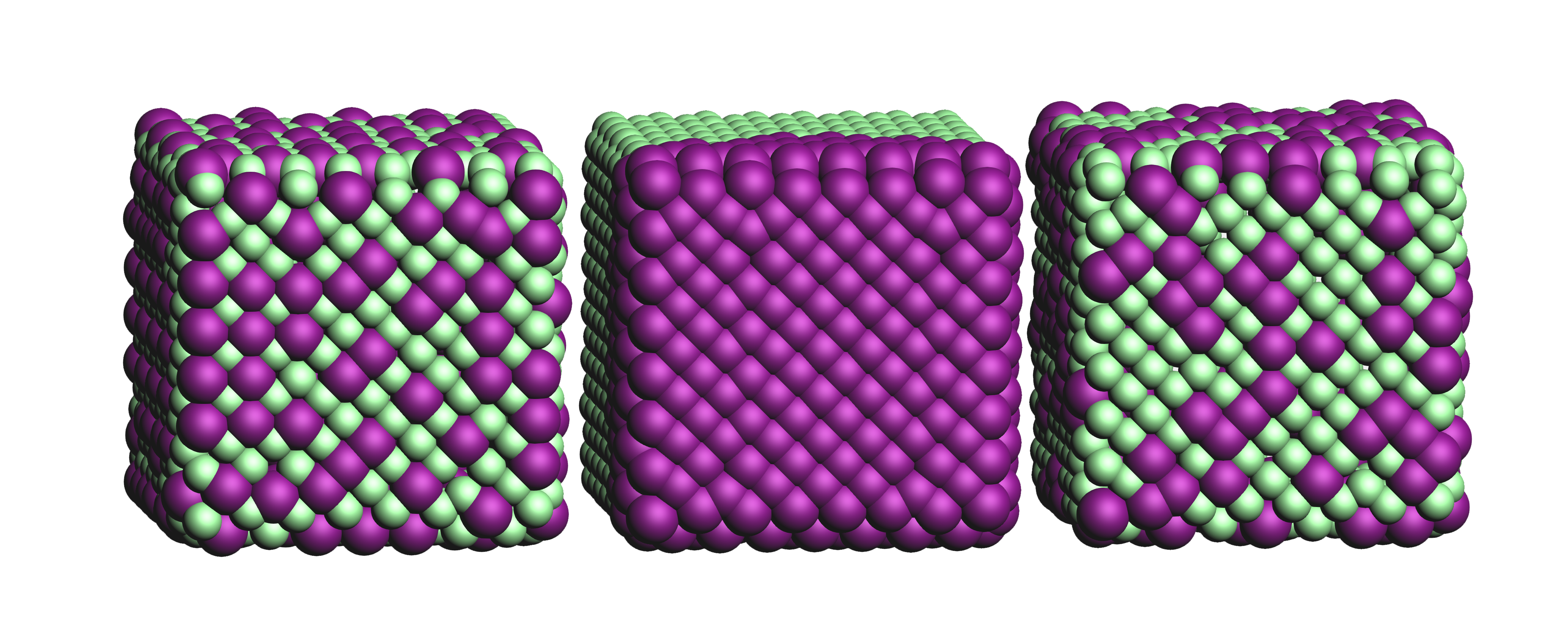Note

An example of four different "uniform" distributions at $$f=1/16$$, $$f=1/8$$, $$f=1/4$$ and $$f=1/2$$.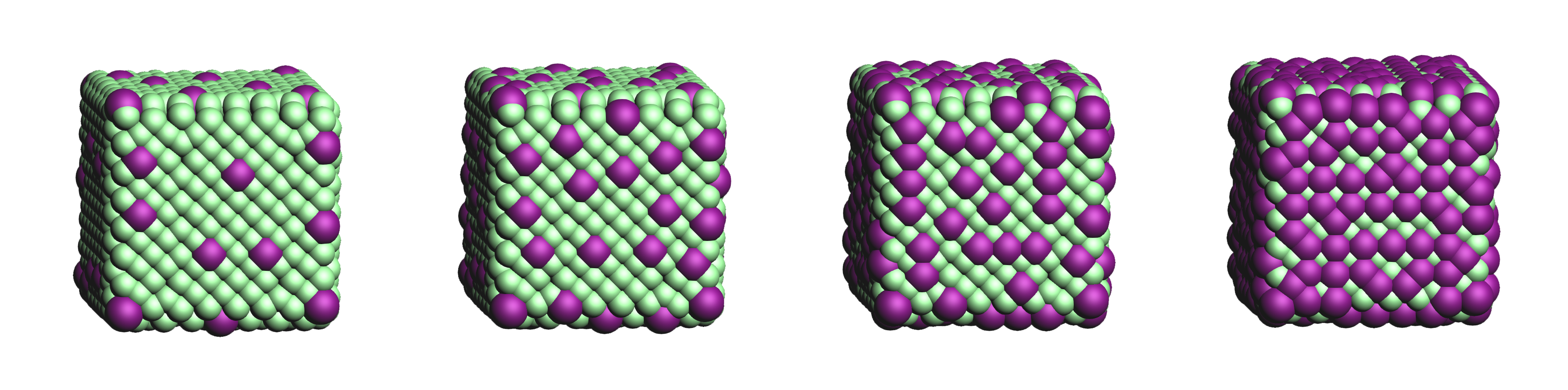optional.core.subset.follow_edge
Parameter

Construct the anchor atom distance matrix by following the shortest path along the edges of a (triangular-faced) polyhedral approximation of the core rather than the shortest path through space.

Enabling this option will result in more accurate "uniform" and "cluster" distributions at the cost of increased computational time.

Given the matrix of Cartesian coordinates $$\boldsymbol{X} \in \mathbb{R}^{n, 3}$$, the matching edge-distance matrix $$\boldsymbol{D}^{\text{edge}} \in \mathbb{R}_{+}^{n, n}$$ and the vector $$\boldsymbol{p} \in \mathbb{N}^{m}$$, representing a (to-be optimized) path as the indices of edge-connected vertices, then element $$D_{i,j}^{\text{edge}}$$ is defined as following:

(3)$D_{i, j}^{\text{edge}} = \min_{\boldsymbol{p} \in \mathbb{N}^{m}; m \in \mathbb{N}} \sum_{k=0}^{m-1} || X_{p_{k},:} - X_{p_{k+1},:} || \quad \text{with} \quad p_{0} = i \quad \text{and} \quad p_{m} = j$

The polyhedron edges are constructed, after projecting all vertices on the surface of a sphere, using Qhull’s ConvexHull algorithm (The Quickhull Algorithm for Convex Hulls). The quality of the constructed edges is proportional to the convexness of the core, more specifically: how well the vertices can be projected on a spherical surface without severely distorting the initial structure. For example, spherical, cylindrical or cuboid cores will yield reasonably edges, while the edges resulting from torus will be extremely poor.

Note

An example of a cores’ polyhedron-representation; displaying the shortest path between points $$i$$ and $$j$$.optional.core.subset.cluster_size
Parameter
• Default value1

Allow for the creation of uniformly distributed clusters of size $$r$$; should be used in conjunction with subset.mode = "uniform".

The value of $$r$$ can be either a single cluster size (e.g. cluster_size = 5) or an iterable of various sizes (e.g. cluster_size = [2, 3, 4]). In the latter case the iterable will be repeated as long as necessary.

Compared to Eq (2) the vector of indices $$\boldsymbol{a} \in \mathbb{N}^{m}$$ is, for the purpose of book keeping, reshaped into the matrix $$\boldsymbol{A} \in \mathbb{N}^{q, r} \; \text{with} \; q*r = m$$. All elements of $$\boldsymbol{A}$$ are, again, constrained to be unique.

(4)$\begin{split}\DeclareMathOperator*{\argmin}{\arg\!\min} A_{i,j} = \begin{cases} \argmin\limits_{k \in \mathbb{N}} \sum_{\hat{\imath}=0}^{n} f \left( D[k, \, \hat{\imath}] \right) & \text{if} & i=0 & \text{and} & j=0 \\ \argmin\limits_{k \in \mathbb{N}} \sum_{\hat{\imath}=0}^{i-1} \sum_{\hat{\jmath}=0}^{r} f \left( D[k, A_{\hat{\imath}, \, \hat{\jmath}}] \right) & \text{if} & i > 0 & \text{and} & j = 0 \\ \argmin\limits_{k \in \mathbb{N}} \dfrac { \sum_{\hat{\imath}=0}^{i-1} \sum_{\hat{\jmath}=0}^{r} f \left( D[k, A_{\hat{\imath}, \, \hat{\jmath}}] \right) } { \sum_{\hat{\jmath}=0}^{j-1} f \left( D[k, A_{i, \, \hat{\jmath}}] \right) } &&& \text{if} & j > 0 \end{cases}\end{split}$

Note

An example of various cluster sizes (1, 2, 3 and 4) with $$f=1/4$$.Note

An example of clusters of varying size (cluster_size = [1, 2, 9, 1]) with $$f=1/4$$.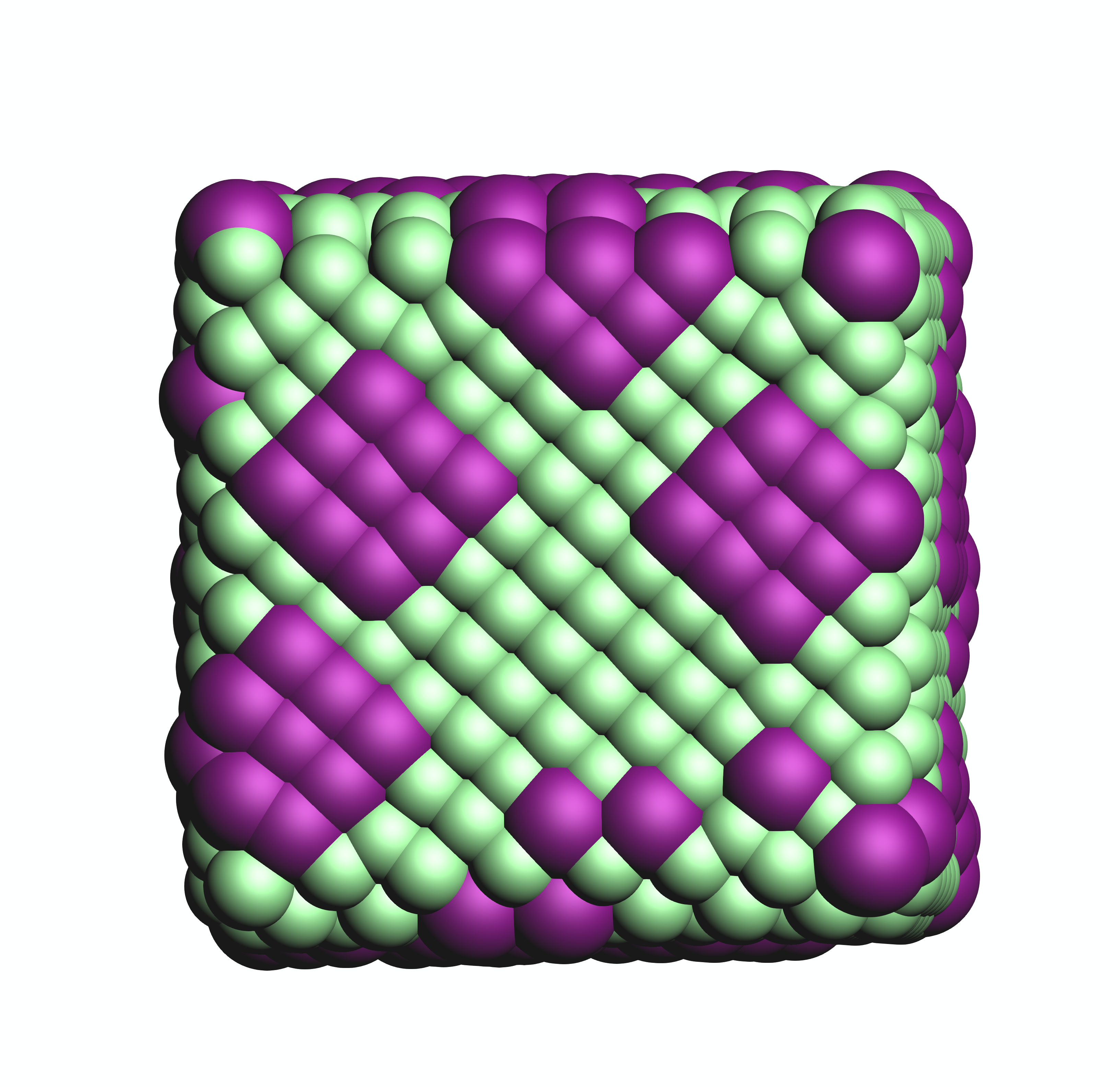optional.core.subset.weight
Parameter

The function $$f(x)$$ for weighting the distance.; its default value corresponds to: $$f(x) = e^{-x}$$.

For the old default, the p-norm with $$p=-2$$, one can use weight = "x**-2": $$f(x) = x^-2$$.

Custom functions can be specified as long as they satisfy the following constraints:

• The function must act an variable by the name of x, a 2D array of positive and/or zero-valued floats ($$x \in \mathbb{R}_{+}^{n, n}$$).

• The function must take a single array as argument and return a new one.

• The function must be able to handle values of numpy.nan and numpy.inf without raising exceptions.

• The shape and data type of the output array should not change with respect to the input.

Modules specified in the weight function will be imported when required, illustrated here with SciPy’s expit function: weight = "scipy.special.expit(x)" aka weight = "1 / (1 + numpy.exp(-x))"

Multi-line statements are allowed: weight = "a = x**2; b = 5 * a; numpy.exp(b)". The last part of the statement is assumed to be the to-be returned value (i.e. return numpy.exp(b)).

optional.core.subset.randomness
Parameter

The probability that each new core anchor atom will be picked at random.

Can be used in combination with "uniform" and "cluster" to introduce a certain degree of randomness (i.e. entropy).

If not None, the provided value should satisfy the following condition: $$0 \le randomness \le 1$$. A value of $$0$$ is equivalent to a "uniform" / "cluster" distribution while $$1$$ is equivalent to "random".

Note

A demonstration of the randomness parameter for a "uniform" and "cluster" distribution at $$f=1/4$$.

The randomness values are (from left to right) set to $$0$$, $$1/4$$, $$1/2$$ and $$1$$.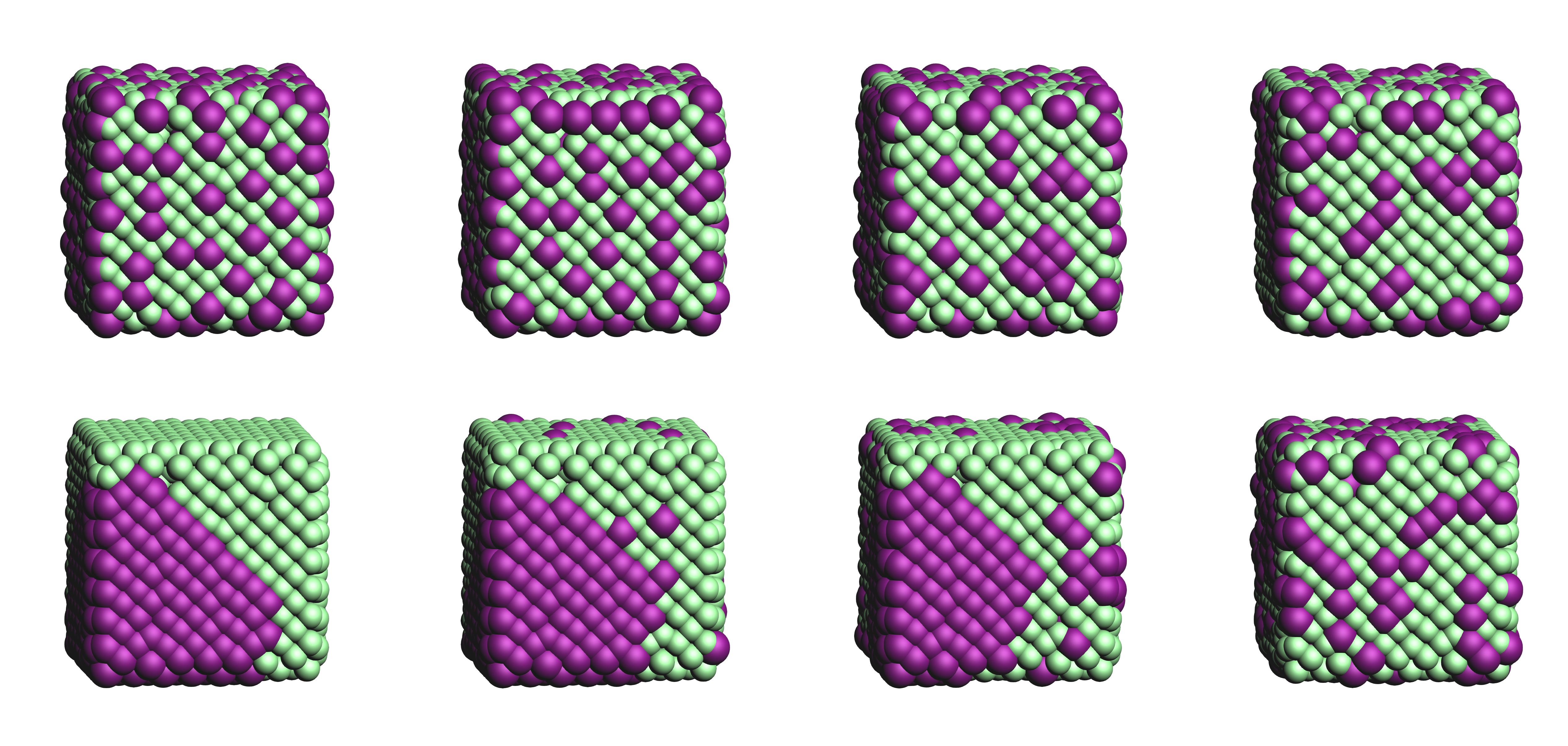### Ligand

optional.ligand

All settings related to the ligands.

Example:

optional:
ligand:
dirname: ligand
optimize: True
anchor: null
split: True
cosmo-rs: False
cdft: False
cone_angle: False


optional.ligand.dirname
Parameter

The name of the directory where all ligands will be stored.

The ligand directory will be created (if it does not yet exist) at the path specified in path.

optional.ligand.optimize
Parameter

Optimize the geometry of the to-be attached ligands.

The ligand is split into one or multiple (more or less) linear fragments, which are subsequently optimized (RDKit UFF [1, 2, 3]) and reassembled while checking for the optimal dihedral angle. The ligand fragments are biased towards more linear conformations to minimize inter-ligand repulsion once the ligands are attached to the core.

After the conformation search a final (unconstrained) geometry optimization is performed, RDKit UFF again being the default level of theory. Custom job types and settings can, respectivelly, be specified with the job2 and s2 keys.

Note

optional:
ligand:
optimize:

optional.ligand.anchor
Parameter
• Default valueNone

Manually specify SMILES strings representing functional groups.

For example, with optional.ligand.anchor = ("O[H]", "[N+].[Cl-]") all ligands will be searched for the presence of hydroxides and ammonium chlorides.

The first atom in each SMILES string (i.e. the “anchor”) will be used for attaching the ligand to the core, while the last atom (assuming optional.ligand.split = True) will be dissociated from the ligand and discarded.

If not specified, the default functional groups of CAT are used.

This option can alternatively be provided as optional.ligand.functional_groups.

Further customization can be achieved by passing dictionaries:

Note

optional:
ligand:
anchor:
- group: "[H]OC(=O)C"  # Remove H and attach at the (formal) oxyanion
group_idx: 1
remove: 0
- group: "[H]OC(=O)C"  # Remove H and attach at the mean position of both oxygens
group_idx: [1, 3]
remove: 0
kind: mean


Note

This argument has no value be default and will thus default to SMILES strings of the default functional groups supported by CAT.

Note

The yaml format uses null rather than None as in Python.

optional.ligand.anchor.group
Parameter

A SMILES string representing the anchoring group.

Note

This argument has no value be default and must thus be provided by the user.

optional.ligand.anchor.group_idx
Parameter

The indices of the anchoring atom(s) in anchor.group.

Indices should be 0-based. These atoms will be attached to the core, the manner in which is determined by the anchor.kind option.

Note

This argument has no value be default and must thus be provided by the user.

optional.ligand.anchor.group_format
Parameter

The format used for representing anchor.group.

Defaults to the SMILES format. The supported formats (and matching RDKit parsers) are as following:

>>> import rdkit.Chem

>>> FASTA      = rdkit.Chem.MolFromFASTA
>>> HELM       = rdkit.Chem.MolFromHELM
>>> INCHI      = rdkit.Chem.MolFromInchi
>>> MOL2       = rdkit.Chem.MolFromMol2Block
>>> MOL2_FILE  = rdkit.Chem.MolFromMol2File
>>> MOL        = rdkit.Chem.MolFromMolBlock
>>> MOL_FILE   = rdkit.Chem.MolFromMolFile
>>> PDB        = rdkit.Chem.MolFromPDBBlock
>>> PDB_FILE   = rdkit.Chem.MolFromPDBFile
>>> PNG        = rdkit.Chem.MolFromPNGString
>>> PNG_FILE   = rdkit.Chem.MolFromPNGFile
>>> SVG        = rdkit.Chem.MolFromRDKitSVG
>>> SEQUENCE   = rdkit.Chem.MolFromSequence
>>> SMARTS     = rdkit.Chem.MolFromSmarts
>>> SMILES     = rdkit.Chem.MolFromSmiles
>>> TPL        = rdkit.Chem.MolFromTPLBlock
>>> TPL_FILE   = rdkit.Chem.MolFromTPLFile

optional.ligand.anchor.remove
Parameter

The indices of the to-be removed atoms in anchor.group.

No atoms are removed when set to None. Indices should be 0-based. See also the split option.

optional.ligand.anchor.kind
Parameter

How atoms are to-be attached when multiple anchor atoms are specified in anchor.group_idx.

Accepts one of the following options:

• "first": Attach the first atom to the core.

• "mean": Attach the mean position of all anchoring atoms to the core.

• "mean_translate": Attach the mean position of all anchoring atoms to the core and then translate back to the first atom.

optional.ligand.anchor.angle_offset
Parameter

Manually offset the angle of the ligand vector by a given number.

The plane of rotation is defined by the first three indices in anchor.group_idx.

By default the angle unit is assumed to be in degrees, but if so desired one can explicitly pass the unit: angle_offset: "0.25 rad".

optional.ligand.anchor.dihedral
Parameter

Manually specify the ligands vector dihedral angle, rather than optimizing it w.r.t. the inter-ligand distance.

The dihedral angle is defined by three vectors:

By default the angle unit is assumed to be in degrees, but if so desired one can explicitly pass the unit: dihedral: "0.5 rad".

optional.ligand.anchor.multi_anchor_filter
Parameter

How ligands with multiple valid anchor sites are to-be treated.

Accepts one of the following options:

• "all": Construct a new ligand for each valid anchor/ligand combination.

• "first": Pick only the first valid functional group, all others are ignored.

• "raise": Treat a ligand as invalid if it has multiple valid anchoring sites.

optional.ligand.split
Parameter

If False: The ligand is to be attached to the core in its entirety .

Before

After

$${NR_4}^+$$

$${NR_4}^+$$

$$O_2 CR$$

$$O_2 CR$$

$$HO_2 CR$$

$$HO_2 CR$$

$$H_3 CO_2 CR$$

$$H_3 CO_2 CR$$

True: A proton, counterion or functional group is to be removed from the ligand before attachment to the core.

Before

After

$$Cl^- + {NR_4}^+$$

$${NR_4}^+$$

$$HO_2 CR$$

$${O_2 CR}^-$$

$$Na^+ + {O_2 CR}^-$$

$${O_2 CR}^-$$

$$HO_2 CR$$

$${O_2 CR}^-$$

$$H_3 CO_2 CR$$

$${O_2 CR}^-$$

optional.ligand.cosmo-rs
Parameter

Perform a property calculation with COSMO-RS [4, 5, 6, 7] on the ligand.

The COSMO surfaces are by default constructed using ADF MOPAC [8, 9, 10].

The solvation energy of the ligand and its activity coefficient are calculated in the following solvents: acetone, acetonitrile, dimethyl formamide (DMF), dimethyl sulfoxide (DMSO), ethyl acetate, ethanol, n-hexane, toluene and water.

optional.ligand.cdft
Parameter

Perform a conceptual DFT (CDFT) calculation with ADF on the ligand.

All global descriptors are, if installed, stored in the database. This includes the following properties:

• Electronic chemical potential (mu)

• Electronic chemical potential (mu+)

• Electronic chemical potential (mu-)

• Electronegativity (chi=-mu)

• Hardness (eta)

• Softness (S)

• Hyperhardness (gamma)

• Electrophilicity index (w=omega)

• Dissocation energy (nucleofuge)

• Dissociation energy (electrofuge)

• Electrodonating power (w-)

• Electroaccepting power(w+)

• Net Electrophilicity

• Global Dual Descriptor Deltaf+

• Global Dual Descriptor Deltaf-

This block can be furthermore customized with one or more of the following keys:

• "keep_files": Whether or not to delete the ADF output afterwards.

• "job1": The type of PLAMS Job used for running the calculation. The only value that should be supplied here (if any) is "ADFJob".

• "s1": The job Settings used for running the CDFT calculation. Can be left blank to use the default template (nanoCAT.cdft.cdft).

Examples

optional:
ligand:
cdft: True

optional:
ligand:
cdft:
s1: ...  # Insert custom settings here

optional.ligand.cone_angle
Parameter

Compute the smallest enclosing cone angle within a ligand.

The smallest enclosing cone angle is herein defined as two times the largest angle ($$2 * \phi_{max}$$) w.r.t. a central ligand vector, the ligand vector in turn being defined as the vector that minimizes $$\phi_{max}$$.

Examples

optional:
ligand:
cone_angle: True

optional:
ligand:
cone_angle:
distance: [0, 0.5, 1, 1.5, 2]

optional.ligand.cone_angle.distance
Parameter
• Default value0.0

The distance in cone_angle of each ligands’ anchor atom w.r.t. the nanocrystal surface.

Accepts one or more distances.

### QD

optional.qd

All settings related to the quantum dots.

Example:

optional:
qd:
dirname: qd
construct_qd: True
optimize: False
bulkiness: False
activation_strain: False
dissociate: False


optional.qd.dirname
Parameter

The name of the directory where all quantum dots will be stored.

The quantum dot directory will be created (if it does not yet exist) at the path specified in path.

optional.qd.construct_qd
Parameter

Whether or not the quantum dot should actually be constructed or not.

Setting this to False will still construct ligands and carry out ligand workflows, but it will not construct the actual quantum dot itself.

optional.qd.optimize
Parameter

Optimize the quantum dot (i.e. core + all ligands) .

By default the calculation is performed with ADF UFF [3, 11]. The geometry of the core and ligand atoms directly attached to the core are frozen during this optimization.

optional.qd.multi_ligand
Parameter

A workflow for attaching multiple non-unique ligands to a single quantum dot.

Note that this is considered a seperate workflow besides the normal ligand attachment. Consequently, these structures will not be passed to further workflows.

See Multi-ligand attachment for more details regarding the available options.

Note

An example with [O-]CCCC as main ligand and [O-]CCCCCCCCCCCCC & [O-]C as additional ligands.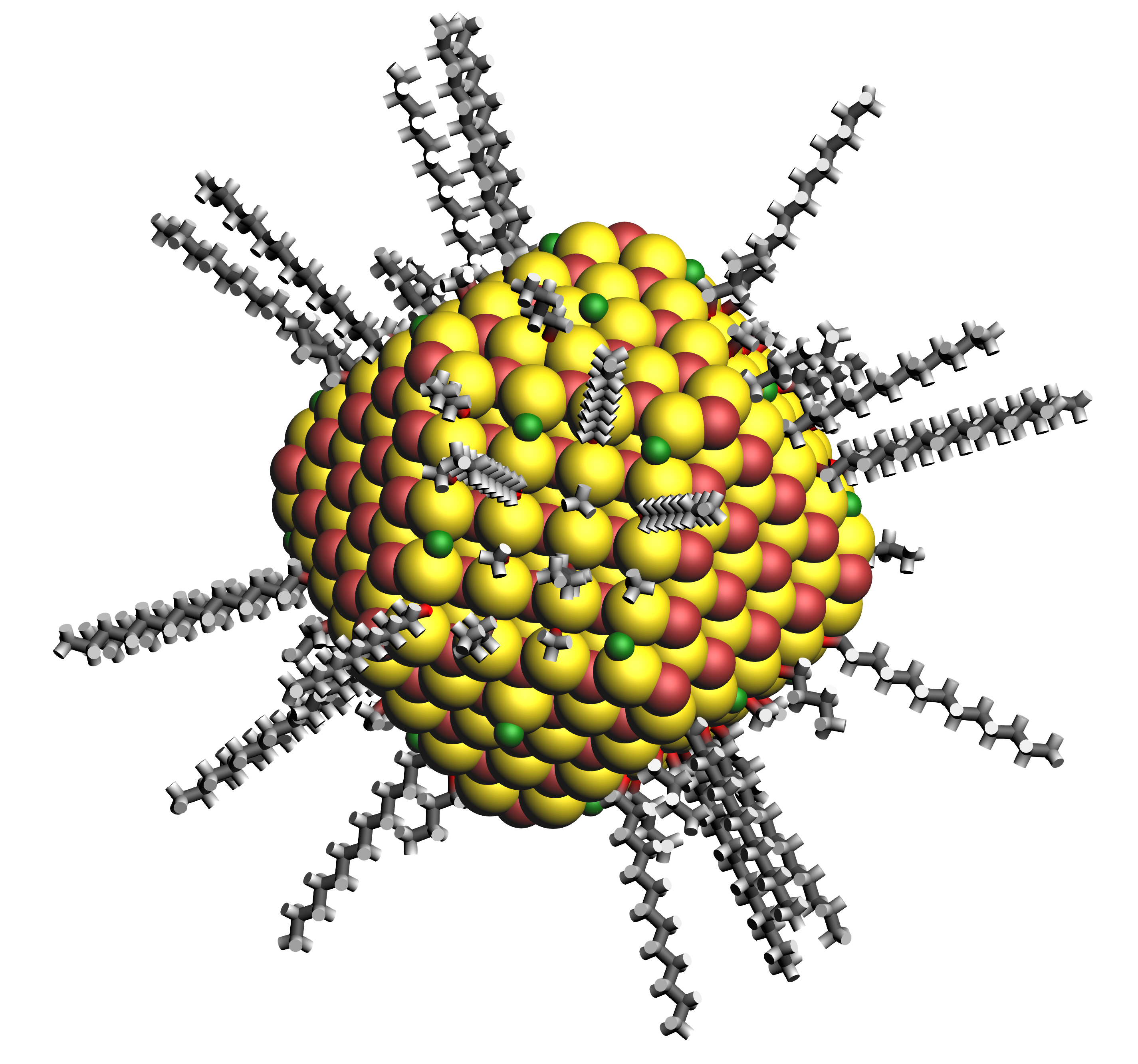optional.qd.bulkiness
Parameter

Calculate the $$V_{bulk}$$, a ligand- and core-specific descriptor of a ligands’ bulkiness.

Supplying a dictionary grants access to the two additional h_lim and d sub-keys.

(5)$V(r_{i}, h_{i}; d, h_{lim}) = \sum_{i=1}^{n} e^{r_{i}} (\frac{2 r_{i}}{d} - 1)^{+} (1 - \frac{h_{i}}{h_{lim}})^{+}$
optional.qd.bulkiness.h_lim
Parameter
• Default value10.0

Default value of the $$h_{lim}$$ parameter in bulkiness.

Set to None to disable the $$h_{lim}$$-based cutoff.

optional.qd.bulkiness.d
Parameter

Default value of the $$d$$ parameter in bulkiness.

Set to "auto" to automatically infer this parameters value based on the mean nearest-neighbor distance among the core anchor atoms. Set to None to disable the $$d$$-based cutoff. Supplying multiple floats will compute the bulkiness for all specified values.

optional.qd.activation_strain
Parameter

Perform an activation strain analysis [12, 13, 14].

The activation strain analysis (kcal mol-1) is performed on the ligands attached to the quantum dot surface with RDKit UFF [1, 2, 3].

The core is removed during this process; the analysis is thus exclusively focused on ligand deformation and inter-ligand interaction. Yields three terms:

1. dEstrain : The energy required to deform the ligand from their equilibrium geometry to the geometry they adopt on the quantum dot surface. This term is, by definition, destabilizing. Also known as the preparation energy (dEprep).

2. dEint : The mutual interaction between all deformed ligands. This term is characterized by the non-covalent interaction between ligands (UFF Lennard-Jones potential) and, depending on the inter-ligand distances, can be either stabilizing or destabilizing.

3. dE : The sum of dEstrain and dEint. Accounts for both the destabilizing ligand deformation and (de-)stabilizing interaction between all ligands in the absence of the core.

See Ensemble-Averaged Activation Strain Analysis for more details.

optional.qd.dissociate
Parameter

Calculate the ligand dissociation energy.

Calculate the ligand dissociation energy (BDE) of ligands attached to the surface of the core. See Bond Dissociation Energy for more details. The calculation consists of five distinct steps:

1. Dissociate all combinations of $${n}$$ ligands ($$Y$$) and an atom from the core ($$X$$) within a radius r from aforementioned core atom. The dissociated compound has the general structure of $$XY_{n}$$.

2. Optimize the geometry of $$XY_{n}$$ at the first level of theory ($$1$$). Default: ADF MOPAC [1, 2, 3].

3. Calculate the “electronic” contribution to the BDE ($$\Delta E$$) at the first level of theory ($$1$$): ADF MOPAC [1, 2, 3]. This step consists of single point calculations of the complete quantum dot, $$XY_{n}$$ and all $$XY_{n}$$-dissociated quantum dots.

4. Calculate the thermochemical contribution to the BDE ($$\Delta \Delta G$$) at the second level of theory ($$2$$). Default: ADF UFF [4, 5]. This step consists of geometry optimizations and frequency analyses of the same compounds used for step 3.

1. $$\Delta G_{tot} = \Delta E_{1} + \Delta \Delta G_{2} = \Delta E_{1} + (\Delta G_{2} - \Delta E_{2})$$.